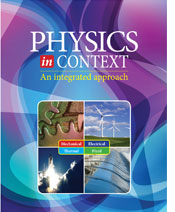# Physics in Context - 2nd Edition

## Chapter 3: RateSome links are repeated for use with more than one lesson.

### 3.1 Rate in Mechanical Systems

Velocity and Acceleration Graphs
http://physics.info/motion-graphs/
This lesson from the Physics Hypertextbook takes the reader through the progression of interpreting graphs of distance, speed, and acceleration. See also links to practice problems and other resources.

Speed and Velocity in One Dimension
http://www.physicsclassroom.com/class/1DKin/Lesson-1/Introduction
This series of pages from the Physics Classroom explains the important role speed and velocity play in analyzing and understanding one-dimensional mechanical systems.

Projectile Motion
http://www.physicsclassroom.com/class/vectors/Lesson-2/What-is-a-Projectile
This series of pages from the Physics Classroom explains the analysis of speed and direction using vectors in two dimensional mechanical systems.

Interesting Properties of Projectile Motion
http://www.phy.ntnu.edu.tw/java/projectile3/projectile3.html
This site includes two applets that 1) examine the effect of different projectile angles fired at the same speed and 2) examine the vector components of a projectile.

Circular Motion
http://www.physicsclassroom.com/class/circles/Lesson-1/Speed-and-Velocity
This series of pages from the Physics Classroom explains the analysis of speed and direction using vectors in two dimensional rotating mechanical systems.

Basic Rotational Variables
http://hyperphysics.phy-astr.gsu.edu/hbase/rotq.html
This page summarizes the relationships between the variables for angles, speeds, and accelerations used in rotating mechanical systems.

Centripetal Force
http://www.physicsclassroom.com/mmedia/circmot/cf.html
Physics Multimedia Studio presentation on Centripetal Force.

The Physics of SUV Rollover Accidents
http://mb-soft.com/public/rollover.html
This presentation will describe the physics (and math) of what occurs, for NON-physicist-types!

Circulation Motion and Centripetal Force
http://www.phy.ntnu.edu.tw/oldjava/circularMotion/circular3D_e.html
An interactive java applet.

### 3.2 Flow in Fluid Systems

Mass Flow Rate
https://www.grc.nasa.gov/www/k-12/airplane/mflow.html
This NASA Glen Research Center page discusses the fundamental concepts related to conservation of energy and mass flow. You can link to additional information on aerodynamics and propulsion.

Movement of Air in Industrial Plants
http://hpac.com/ventilation-iaq/movement_air_industrial
This article at the Heating, Piping, and Air Conditioning (HPAC) Engineering site discusses how an analysis of air flow, as impacted by mass and density, was sued to solve a real-world manufacturing problem.

Continuity and Conservation of Matter
http://www.efm.leeds.ac.uk/CIVE/CIVE1400/Section3/continuity.htm
This page from a fluid mechanics course uses diagrams, mathematics, and example problems to aid in the understanding of mass flow rate and volume flow rate.

Fluids in Motion
http://theory.uwinnipeg.ca/physics/fluids/node11.html
This page defines flow rate and then derives the continuity equation and Bernouilli's equation.

### 3.3 Current in Electrical Systems

Electric Current
http://physics.info/electric-current/
This lesson from the Physics Hypertextbook presents the concept of electric current by showing how the movement of electric charge through a conductor, and includes some interesting historical perspectives. Some of the material may be more advanced than necessary for this course.

AC Waveforms
http://www.sweethaven.com/sweethaven/ModElec/acee/aceeTOC.asp
This page links to several lessons in a unit on "AC Waveforms" that introduce the sine-waves used to define AC electricity. This page links to several lessons in a unit on "AC Waveforms" that introduce the sine-waves used to define AC electricity. Click the links for lessons in "Unit 1 AC Waveforms."

This site includes a series of pages explaining schematic symbols used to describe electronic circuits.

Electric Current
http://www.physicsclassroom.com/class/circuits/Lesson-2/What-is-an-Electric-Circuit
This series of lessons from the Physics Classroom discusses how electric potential causes electric charges to move through a circuit. After completing the practice problems in each lesson, click "Next Section" to advance.

Experiments in Electricity
http://www.stevespanglerscience.com/lab/experiments/build-a-light-bulb-circuit-science
This page describes an activity to construct and test a light bulb, including a video demonstration.

### 3.4 Heat in Thermal Systems

http://eo.ucar.edu/skymath/tmp2.html
This page delivers an intermediate level discussion of temperature, thermometers, the kinetic theory of gas, and thermal radiation.

Heat and Temperature
http://www.concord.org/HOP/htu/htu.concepts.flow.html